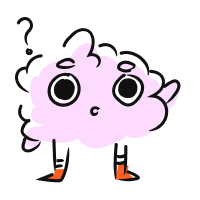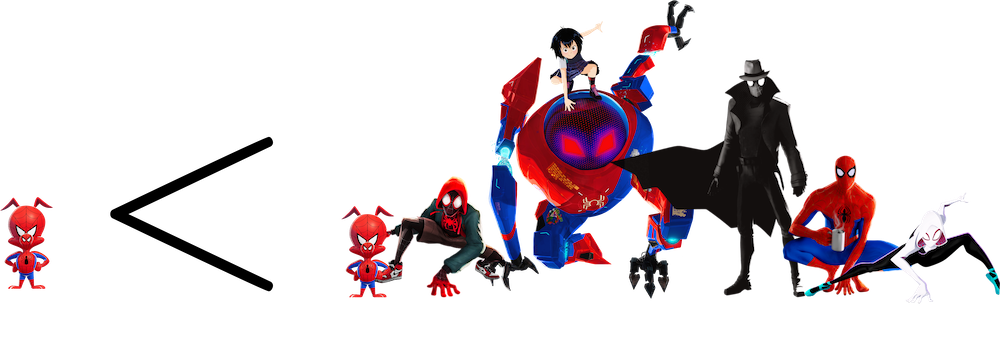STUDENT
TEACHER

Greater Than and Less Than Calculator

STUDENT
TEACHER

Greater Than and Less Than Calculator

# Greater Than and Less Than: Comparing Decimals

CALCULATOR

## Greater Than and Less Than Calculator

Compare
andWe have some questions for you! Help us out through this
INTRO

Remembering which inequality sign or symbol to use in a number comparison can be difficult, but we have an easy memory trick that can help!

See how the inequality symbol has a wide end and a narrow end?The wide end opens to the greater value, and the narrow end points to the lesser value. If we describe the image below from left to right, it reads as all the Spider People are greater (or bigger) than just one Spider-Ham.If we flip things around, we can still read it left to right, but now it reads as one Spider-Ham is less (or smaller) than all the Spider People.We can apply the same concept to comparing numbers. The wide end of the sign should always open to the greater number.
KEY STEPS

## How to Compare Numbers

Compare a

### Step 2. Compare digits from left to right.

• If they’re the same, move to the next digit.
• If they’re different, the bigger digit belongs to the number that is farther from 0.

### Step 3. Imagine the two decimals on a number line.

The number farthest to the right is the greater number.

### Step 4. Use the correct inequality sign.

The wide end of the sign should always open to the greater number.
LESSON
Comparing Decimals
PRACTICE
Comparing Decimals
CONCLUSION
Leave Feedback